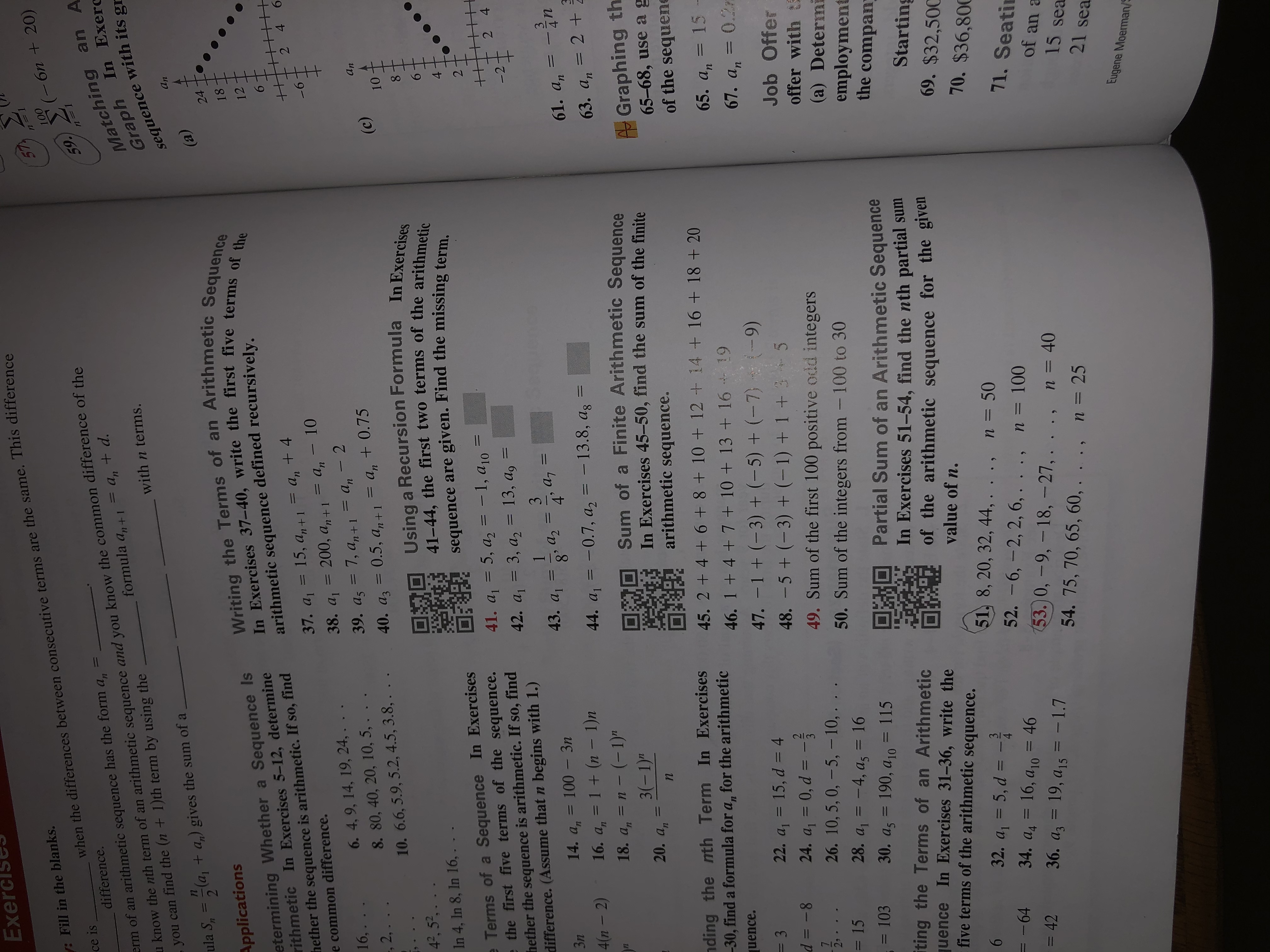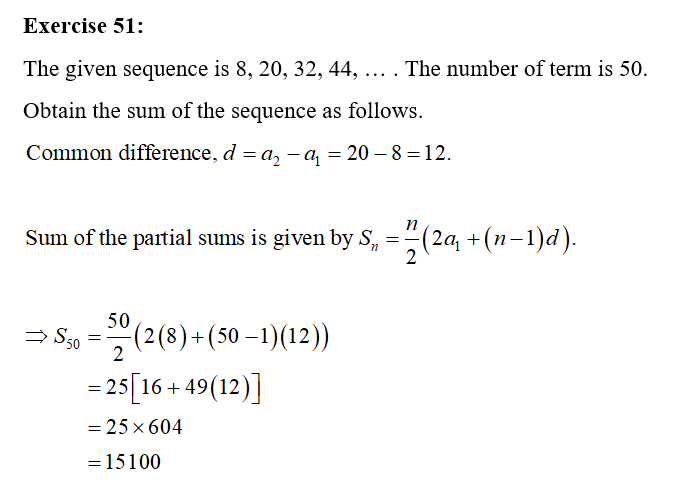# Exel: Fill in the blanks.57100(-6n + 20)when the differences between consecutive terms are the same. This differencece isdifference.u know the nth term of an arithmetic sequence and you know the common difference of the-you can find the (n + 1)th term by using the%3DMatching an AGraph In Exercsequence with its gr5917+ d.erm of an arithmetic sequence has the form= Anformula a, + 1with n terms.la,ula S, = (a, + a,) gives the sum of a%3Dan(a)Writing the Terms of an Arithmetic SequenceIn Exercises 37-40, write the first five terms of theApplications24etermining Whether a Sequence Isrithmetic In Exercises 5-12, determinehether the sequence is arithmetic. If so, finde common difference.1812arithmetic sequence defined recursively.An37. a, = 15, a, +1 = a, + 4200, a, +1 = a, – 106.2 4= Un9-38. a16. 4, 9, 14, 19, 24, . .8. 80, 40, 20, 10, 5, . .16,.39. az = 7, a, +1 = a, - 20.5, an+1..= Un%3D-, 2, . . .Anan40. az = 0.5, a,+1 = a, + 0.75%3D10. 6.6, 5.9, 5.2, 4.5, 3.8, . . .(c)Using a Recursion Formula In Exercises41-44, the first two terms of the arithmeticsequence are given. Find the missing term.104°, 52,. . .In 4, In 8, In 16, ...6.e Terms of a Sequence In Exercisese the first five terms of the sequence.hether the sequence is arithmetic. If so, finddifference. (Assume that n begins with 1.)41. a = 5, a, = -1, a1042. a, = 3, a, = 13, a, =2%3D2 4-23143. a1a7%3Da2814. а, — 100 — ЗпЗп4%3D316. a, = 1 + (n – 1)n18. a, = n - (-1)"3(-1)"61. an4(n – 2)%3D44. a, = -0.7, a, = - 13.8, ag%3D63. a, = 2 +Sum of a Finite Arithmetic Sequence*In Exercises 45-50, find the sum of the finitearithmetic sequence.20. anGraphing th.65-68, use agof the sequenending the nth Term In Exercises-30, find a formula for a, for the arithmetic45. 2 + 4 + 6 + 8 + 10 + 12 + 14 + 16 + 18 + 2065. an46. 1 + 4 + 7 + 10 + 13+ 16 + 1915uence.%3D47. -1 + (-3) + (-5) + (-7)67. an0.2m(-9)22. a, = 15, d = 424. a, = 0, d = -26. 10, 5, 0, – 5, – 10,. . .48. - 5 + (-3) + (-1) + 1 + 3- 5d = -8Job Offeroffer with t(a) Determemploymentthe compan%3D%3D349. Sum of the first 100 positive odd integers250. Sum of the integers from -100 to 30= 1528. a, = -4, a, = 16%3D= 10330. a, = 190, a10 = 115OROPartial Sum of an Arithmetic SequenceIn Exercises 51-54, find the nth partial sumof the arithmetic sequence for the givenvalue of n.%3Diting the Terms of an Arithmeticquence In Exercises 31-36, write thefive terms of the arithmetic sequence.Starting69. $32,5006.51, 8, 20, 32, 44, . . ., n = 5052. - 6, — 2, 2, 6, . .., п%3D 10053.0,-9,-18,-27,. . ., n= 4054. 75, 70, 65, 60, . . ., n = 2570.$36,80032. a, = 5, d = -3%3D-6434. a, = 16, a10 = 46%3D71. Seatin= 4236. az = 19, a,5 = -1.7%3Dof an a%3D15 sea21 seaEugene Moerman/S64-

Question
1 views

How do you answer 51 and 53?

(This is not graded but, rather, an exercise to achieve acquisition).help_outlineImage TranscriptioncloseExel : Fill in the blanks. 57 100 (-6n + 20) when the differences between consecutive terms are the same. This difference ce is difference. u know the nth term of an arithmetic sequence and you know the common difference of the -you can find the (n + 1)th term by using the %3D Matching an A Graph In Exerc sequence with its gr 59 17 + d. erm of an arithmetic sequence has the form = An formula a, + 1 with n terms. la, ula S, = (a, + a,) gives the sum of a %3D an (a) Writing the Terms of an Arithmetic Sequence In Exercises 37-40, write the first five terms of the Applications 24 etermining Whether a Sequence Is rithmetic In Exercises 5-12, determine hether the sequence is arithmetic. If so, find e common difference. 18 12 arithmetic sequence defined recursively. An 37. a, = 15, a, +1 = a, + 4 200, a, +1 = a, – 10 6. 2 4 = Un 9- 38. a1 6. 4, 9, 14, 19, 24, . . 8. 80, 40, 20, 10, 5, . . 16,. 39. az = 7, a, +1 = a, - 2 0.5, an+1 . . = Un %3D -, 2, . . . An an 40. az = 0.5, a,+1 = a, + 0.75 %3D 10. 6.6, 5.9, 5.2, 4.5, 3.8, . . . (c) Using a Recursion Formula In Exercises 41-44, the first two terms of the arithmetic sequence are given. Find the missing term. 10 4°, 52,. . . In 4, In 8, In 16, ... 6. e Terms of a Sequence In Exercises e the first five terms of the sequence. hether the sequence is arithmetic. If so, find difference. (Assume that n begins with 1.) 41. a = 5, a, = -1, a10 42. a, = 3, a, = 13, a, = 2 %3D 2 4 -2 3 1 43. a1 a7 %3D a2 8 14. а, — 100 — Зп Зп 4 %3D 3 16. a, = 1 + (n – 1)n 18. a, = n - (-1)" 3(-1)" 61. an 4(n – 2) %3D 44. a, = -0.7, a, = - 13.8, ag %3D 63. a, = 2 + Sum of a Finite Arithmetic Sequence *In Exercises 45-50, find the sum of the finite arithmetic sequence. 20. an Graphing th. 65-68, use ag of the sequene nding the nth Term In Exercises -30, find a formula for a, for the arithmetic 45. 2 + 4 + 6 + 8 + 10 + 12 + 14 + 16 + 18 + 20 65. an 46. 1 + 4 + 7 + 10 + 13+ 16 + 19 15 uence. %3D 47. -1 + (-3) + (-5) + (-7) 67. an 0.2m (-9) 22. a, = 15, d = 4 24. a, = 0, d = - 26. 10, 5, 0, – 5, – 10,. . . 48. - 5 + (-3) + (-1) + 1 + 3- 5 d = -8 Job Offer offer with t (a) Determ employment the compan %3D %3D 3 49. Sum of the first 100 positive odd integers 2 50. Sum of the integers from -100 to 30 = 15 28. a, = -4, a, = 16 %3D = 103 30. a, = 190, a10 = 115 ORO Partial Sum of an Arithmetic Sequence In Exercises 51-54, find the nth partial sum of the arithmetic sequence for the given value of n. %3D iting the Terms of an Arithmetic quence In Exercises 31-36, write the five terms of the arithmetic sequence. Starting 69. $32,500 6. 51, 8, 20, 32, 44, . . ., n = 50 52. - 6, — 2, 2, 6, . .., п%3D 100 53.0,-9,-18,-27,. . ., n= 40 54. 75, 70, 65, 60, . . ., n = 25 70.$36,800 32. a, = 5, d = - 3 %3D -64 34. a, = 16, a10 = 46 %3D 71. Seatin = 42 36. az = 19, a,5 = -1.7 %3D of an a %3D 15 sea 21 sea Eugene Moerman/S 6 4- fullscreen
check_circle

Step 1...

### Want to see the full answer?

See Solution

#### Want to see this answer and more?

Solutions are written by subject experts who are available 24/7. Questions are typically answered within 1 hour.*

See Solution
*Response times may vary by subject and question.
Tagged in